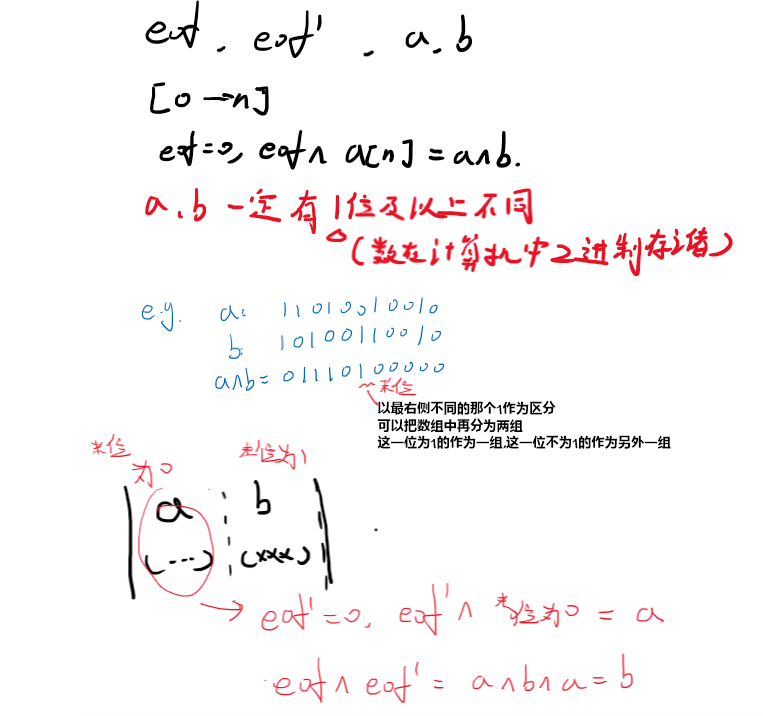1.异或的本质是 无进位相加->相同为0,不同为1
2.异或的性质 a^a=0, a^0=a 以及交换律,结合率

1.不占用额外空间的交换位置a<->b

``````a=a^b;
b=a^b;
a=a^b;
``````2.一个数组中一个数出现奇数次,其他数出现偶数次,通过异或找到该奇数次的数

``````[伪代码]
a[n];
auto eor=0;
for(i from 0 to n)eor=eor^a[i];
``````

3.一个数组中两个数出现奇数次,其他数出现偶数次,也可以通过异或找到该奇数次的数(进阶)``````[伪代码]
a[n];
int eor=0,eor`=0;
for(i from 0 to n)eor=eor^a[i];

int RightOne=  eor&(~eor+1);//得到一个筛选数,该数可以筛选出第X位为1的数
for(int cur:a[n])
if(a[n]&RightOne!=0)eor`=(eor`)^a[n];//此时第X为1的数被异或进eor`,但偶数次数的相抵消为0,eor`存的是a或者b中第X位为1的那个数

eof=eof^eof`;//a^b^a=b,另一个数.即eof和eof`分别保存了两个次数为1的数
``````